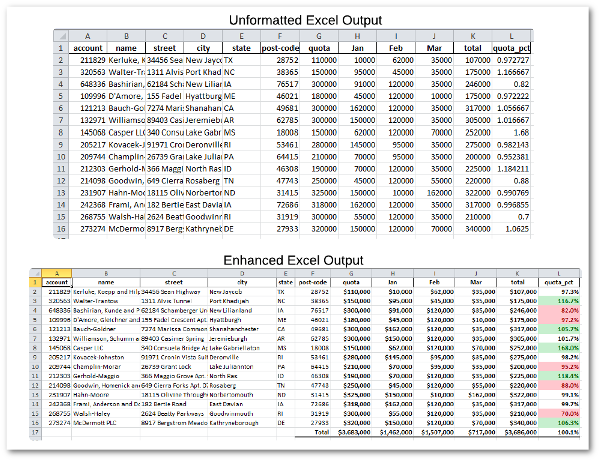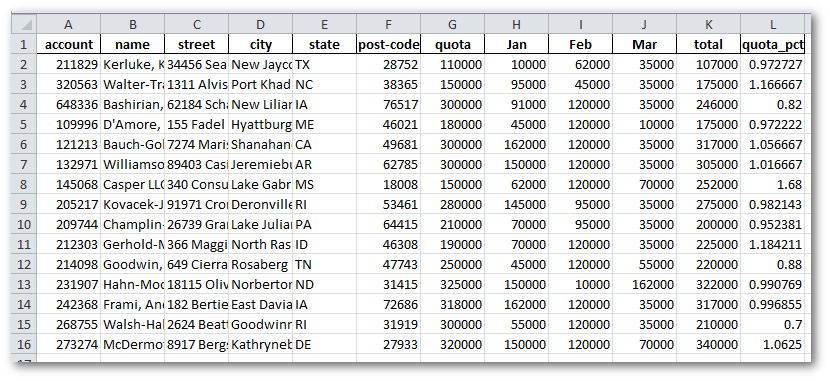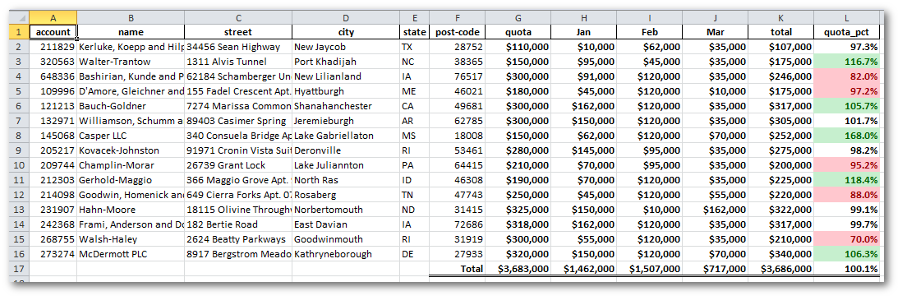Taking care of business, one python script at a time

Wed 08 April 2015

# Improving Pandas’s Excel Output

Posted by Chris Moffitt in articles

## Introduction

Pandas makes it very easy to output a DataFrame to Excel. However, there are limited options for customizing the output and using Excel’s features to make your output as useful as it could be. Fortunately, it is easy to use the excellent XlsxWriter module to customize and enhance the Excel workbooks created by Panda’s ``` to_excel``` function. This article will describe how to use XlsxWriter and Pandas to make complex, visually appealing and useful Excel workbooks. As an added bonus, the article will briefly discuss the use of the new ``` assign``` function that has been introduced in pandas 0.16.0.

Since this is a discussion about making Excel look better, a visual will probably be a quicker way to show what we can accomplish with a little bit of extra python code on top of the standard pandas library.## Background

If you are not familiar with pandas and how to use it to manipulate data, some of these prior articles might put it in perspective:

One other point to clarify is that you must be using pandas 0.16 or higher to use ``` assign``` . In addition there was a subtle bug in prior pandas versions that would not allow the formatting to work correctly when using XlsxWriter as shown below.

Version Warning
Please make sure you have a version of pandas ( > 0.16) installed for this example to work.

For the purposes of this article, I will be using data very similar to the ones described in Common Excel Tasks Demonstrated in Pandas.

## Simple Output

Let’s get started by importing pandas, numpy and ``` xl_rowcol_to_cell``` .

```import pandas as pd
import numpy as np
from xlsxwriter.utility import xl_rowcol_to_cell
```

```df = pd.read_excel("excel-comp-datav2.xlsx")
```
account name street city state post-code quota Jan Feb Mar
0 211829 Kerluke, Koepp and Hilpert 34456 Sean Highway New Jaycob TX 28752 110000 10000 62000 35000
1 320563 Walter-Trantow 1311 Alvis Tunnel Port Khadijah NC 38365 150000 95000 45000 35000
2 648336 Bashirian, Kunde and Price 62184 Schamberger Underpass Apt. 231 New Lilianland IA 76517 300000 91000 120000 35000
3 109996 D’Amore, Gleichner and Bode 155 Fadel Crescent Apt. 144 Hyattburgh ME 46021 180000 45000 120000 10000
4 121213 Bauch-Goldner 7274 Marissa Common Shanahanchester CA 49681 300000 162000 120000 35000

This dummy data shows account sales for Jan, Feb and March as well as the quota for each of these accounts. What we are going to do is summarize the data and see how close each account was towards hitting its quota.

Get the number of rows to make it easier to add our Excel formulas a little later.

```number_rows = len(df.index)
```

As of pandas 0.16, there is a new function called ``` assign``` that is useful here to add some total data.

```# Add some summary data using the new assign functionality in pandas 0.16
df = df.assign(total=(df['Jan'] + df['Feb'] + df['Mar']))
```
account name street city state post-code quota Jan Feb Mar total
0 211829 Kerluke, Koepp and Hilpert 34456 Sean Highway New Jaycob TX 28752 110000 10000 62000 35000 107000
1 320563 Walter-Trantow 1311 Alvis Tunnel Port Khadijah NC 38365 150000 95000 45000 35000 175000
2 648336 Bashirian, Kunde and Price 62184 Schamberger Underpass Apt. 231 New Lilianland IA 76517 300000 91000 120000 35000 246000
3 109996 D’Amore, Gleichner and Bode 155 Fadel Crescent Apt. 144 Hyattburgh ME 46021 180000 45000 120000 10000 175000
4 121213 Bauch-Goldner 7274 Marissa Common Shanahanchester CA 49681 300000 162000 120000 35000 317000

We can also use ``` assign``` to show how close accounts are towards their quota.

```df = df.assign(quota_pct=(1+(df['total'] - df['quota'])/df['quota']))
```
account name street city state post-code quota Jan Feb Mar total quota_pct
0 211829 Kerluke, Koepp and Hilpert 34456 Sean Highway New Jaycob TX 28752 110000 10000 62000 35000 107000 0.972727
1 320563 Walter-Trantow 1311 Alvis Tunnel Port Khadijah NC 38365 150000 95000 45000 35000 175000 1.166667
2 648336 Bashirian, Kunde and Price 62184 Schamberger Underpass Apt. 231 New Lilianland IA 76517 300000 91000 120000 35000 246000 0.820000
3 109996 D’Amore, Gleichner and Bode 155 Fadel Crescent Apt. 144 Hyattburgh ME 46021 180000 45000 120000 10000 175000 0.972222
4 121213 Bauch-Goldner 7274 Marissa Common Shanahanchester CA 49681 300000 162000 120000 35000 317000 1.056667

Thoughts on using assign

As a side note, I personally like the ``` assign``` function for adding these types of additional columns. Here is how you could add a total without assign:

```df["total"] = df["Jan"] + df["Feb"] + df["Mar"]
```

Save this data using the simple ``` to_excel``` process.

```writer_orig = pd.ExcelWriter('simple.xlsx', engine='xlsxwriter')
df.to_excel(writer_orig, index=False, sheet_name='report')
writer_orig.save()
```

Here is what the simple file looks like:You will notice a couple of things that would be nice to fix:

• The column widths make it tough to see all the data
• The sales and percentages are not formmatted as dollars or %’s
• There is no total information.
• Overall it is pretty boring.

## Complex Output

With just a little more coding, we can create a more sophisticated output.

We create another writer and use the ``` to_excel``` to create our workbook.

```writer = pd.ExcelWriter('fancy.xlsx', engine='xlsxwriter')
df.to_excel(writer, index=False, sheet_name='report')
```

The key is to get access to the worksheet which enables us to use all the XlsxWriter capability available in that library.

```workbook = writer.book
worksheet = writer.sheets['report']
```

Now that we have the worksheet, we can do anything that xlsxwriter supports. If you have not done so yet, I encourage you to take a look at the XlsxWriter docs. They are very well written and show you all the capabilities available for customizing Excel output. By accessing the worksheet as shown above, you can easily drop in all XlsxWriter’s features into your output.

I’ll walk through a few of them to give you some ideas. First, we resize the sheet by adjusting the zoom.

```worksheet.set_zoom(90)
```

Some of our biggest improvements come through formatting the columns to make the data more readable. ``` add_format``` is very useful for improving your standard output.

Here are two examples of formatting numbers:

```# Add a number format for cells with money.
money_fmt = workbook.add_format({'num_format': '\$#,##0', 'bold': True})

# Add a percent format with 1 decimal point
percent_fmt = workbook.add_format({'num_format': '0.0%', 'bold': True})
```

```# Total formatting
total_fmt = workbook.add_format({'align': 'right', 'num_format': '\$#,##0',
'bold': True, 'bottom':6})
# Total percent format
total_percent_fmt = workbook.add_format({'align': 'right', 'num_format': '0.0%',
'bold': True, 'bottom':6})
```

Change the size of several columns using ``` set_column``` . This can also be used to apply formatting to a column.

This section changes the size of columns B-F to sizes more appropriate for the data they store.

```# Account info columns
worksheet.set_column('B:D', 20)
# State column
worksheet.set_column('E:E', 5)
# Post code
worksheet.set_column('F:F', 10)
```

In addition to changing the size of G-L, we also can apply the money and percent formatting on the entire column.

```# Monthly columns
worksheet.set_column('G:K', 12, money_fmt)
# Quota percent columns
worksheet.set_column('L:L', 12, percent_fmt)
```

The next section adds a total at the bottom of our data. The biggest challenge in working with Excel is converting between numeric indices and cell labels. This loop shows how to loop through the columns numerically but also use ``` xl_rowcol_to_cell``` to get cell locations.

```# Add total rows
for column in range(6, 11):
# Determine where we will place the formula
cell_location = xl_rowcol_to_cell(number_rows+1, column)
# Get the range to use for the sum formula
start_range = xl_rowcol_to_cell(1, column)
end_range = xl_rowcol_to_cell(number_rows, column)
# Construct and write the formula
formula = "=SUM({:s}:{:s})".format(start_range, end_range)
worksheet.write_formula(cell_location, formula, total_fmt)
```

In addition to writing the total label, we want to see what our % to quota is in aggregate. We construct the string to calculate the percent to quota and write it out using ``` write_formula```

```# Add a total label
worksheet.write_string(number_rows+1, 5, "Total",total_fmt)
percent_formula = "=1+(K{0}-G{0})/G{0}".format(number_rows+2)
worksheet.write_formula(number_rows+1, 11, percent_formula, total_percent_fmt)
```

The final item to add is the capability to highlight the top 5 values and the bottom 5 values. This gives us a nice visual representation of where we may need to inspect further.

First, we define the range we want to format.

```# Define our range for the color formatting
color_range = "L2:L{}".format(number_rows+1)
```

Then, we define the colors.

```# Add a format. Light red fill with dark red text.
'font_color': '#9C0006'})

# Add a format. Green fill with dark green text.
'font_color': '#006100'})
```

Finally, we apply the conditional formatting and save our output.

```# Highlight the top 5 values in Green
worksheet.conditional_format(color_range, {'type': 'top',
'value': '5',
'format': format2})
```
```# Highlight the bottom 5 values in Red
worksheet.conditional_format(color_range, {'type': 'bottom',
'value': '5',
'format': format1})
writer.save()
```

Here is the final output.## Final Thoughts

To pull it all together, here is the complete code:

```"""
Show examples of modifying the Excel output generated by pandas
"""
import pandas as pd
import numpy as np

from xlsxwriter.utility import xl_rowcol_to_cell

# We need the number of rows in order to place the totals
number_rows = len(df.index)

# Add some summary data using the new assign functionality in pandas 0.16
df = df.assign(total=(df['Jan'] + df['Feb'] + df['Mar']))
df = df.assign(quota_pct=(1+(df['total'] - df['quota'])/df['quota']))

# Create a Pandas Excel writer using XlsxWriter as the engine.
# Save the unformatted results
writer_orig = pd.ExcelWriter('simple.xlsx', engine='xlsxwriter')
df.to_excel(writer_orig, index=False, sheet_name='report')
writer_orig.save()

# Create a Pandas Excel writer using XlsxWriter as the engine.
writer = pd.ExcelWriter('fancy.xlsx', engine='xlsxwriter')
df.to_excel(writer, index=False, sheet_name='report')

workbook = writer.book
worksheet = writer.sheets['report']

# Reduce the zoom a little
worksheet.set_zoom(90)

# Add a number format for cells with money.
money_fmt = workbook.add_format({'num_format': '\$#,##0', 'bold': True})

# Add a percent format with 1 decimal point
percent_fmt = workbook.add_format({'num_format': '0.0%', 'bold': True})

# Total formatting
total_fmt = workbook.add_format({'align': 'right', 'num_format': '\$#,##0',
'bold': True, 'bottom':6})
# Total percent format
total_percent_fmt = workbook.add_format({'align': 'right', 'num_format': '0.0%',
'bold': True, 'bottom':6})

# Format the columns by width and include number formats

# Account info columns
worksheet.set_column('B:D', 20)
# State column
worksheet.set_column('E:E', 5)
# Post code
worksheet.set_column('F:F', 10)

# Monthly columns
worksheet.set_column('G:K', 12, money_fmt)
# Quota percent columns
worksheet.set_column('L:L', 12, percent_fmt)

for column in range(6, 11):
# Determine where we will place the formula
cell_location = xl_rowcol_to_cell(number_rows+1, column)
# Get the range to use for the sum formula
start_range = xl_rowcol_to_cell(1, column)
end_range = xl_rowcol_to_cell(number_rows, column)
# Construct and write the formula
formula = "=SUM({:s}:{:s})".format(start_range, end_range)
worksheet.write_formula(cell_location, formula, total_fmt)

worksheet.write_string(number_rows+1, 5, "Total",total_fmt)
percent_formula = "=1+(K{0}-G{0})/G{0}".format(number_rows+2)
worksheet.write_formula(number_rows+1, 11, percent_formula, total_percent_fmt)

# Define our range for the color formatting
color_range = "L2:L{}".format(number_rows+1)

# Add a format. Light red fill with dark red text.
'font_color': '#9C0006'})

# Add a format. Green fill with dark green text.
'font_color': '#006100'})

# Highlight the top 5 values in Green
worksheet.conditional_format(color_range, {'type': 'top',
'value': '5',
'format': format2})

# Highlight the bottom 5 values in Red
worksheet.conditional_format(color_range, {'type': 'bottom',
'value': '5',
'format': format1})

writer.save()
```

There is much more to explore but this quick overview should give you some good ideas about making pandas’ Excel output that much more useful.